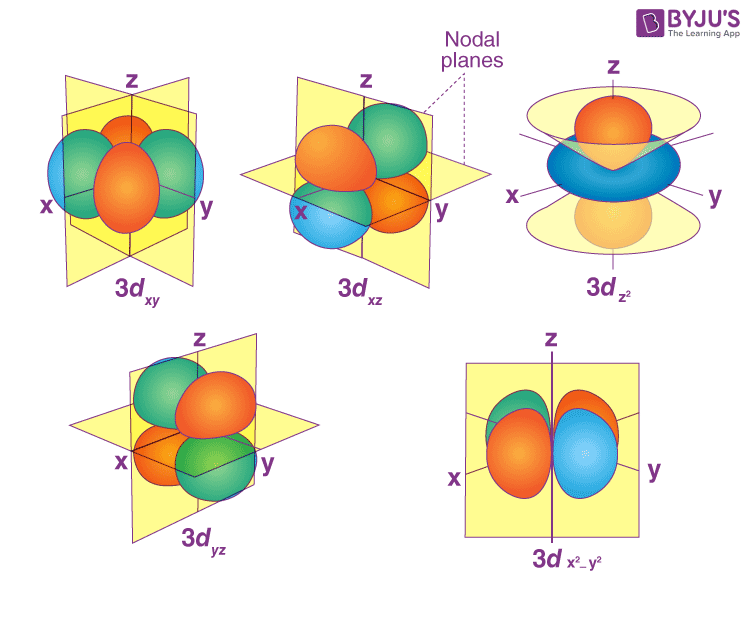Defend yourself better by mastering the science of immunity and vaccines. Secure your free spot, now! Defend yourself better by mastering the science of immunity and vaccines. Secure your free spot, now!

# Radial and Angular nodes formula

## What are radial and angular nodes?

The regions or spaces around the nucleus where the probability of finding an electron is zero are called nodes.

The atomic orbitals or orbital wave functions can be represented by the product of two wave functions, radial and angular wave function. A node is a point where a wave function passes through zero. The nodes are classified into two types (i) Radial nodes and (ii) Angular nodes.

The spherical surfaces around the nucleus where the probability of finding an electron is zero are called radial nodes. The planes or planar areas around the nucleus where the probability of finding an electron is zero are called angular nodes.Radial nodes are the spherical surface region where the probability of finding an electron is zero. It depends on both the values of the principal quantum number and the azimuthal quantum number. The number of nodes of particular orbital an increases with increase in their principal quantum number.

Radial nodes can be find out by the formula

Number of Radial nodes  =  n-l-1 = n-(l+1)

Where  n = principal quantum number, l = Azimuthal quantum number

### (a) Calculating the number of radial nodes of 1s orbital;

In 1s orbital, the value of principal quantum number (n)= 1 and the value of Azimuthal quantum number (l)= 0

Number of Radial nodes = n – l -1 = 1-0-1= 0

### (b) Calculating the number of radial nodes of 2s orbital;

In 1s orbital, the value of principal quantum number (n)= 2 and the value of Azimuthal quantum number (l)= 0

Number of Radial nodes = n – l-1= 2-0-1= 1

### (c)  Calculating the number of radial nodes of 3s orbital;

In 1s orbital, the value of principal quantum number (n)= 3 and the value of Azimuthal quantum number (l)= 0

Number of Radial nodes = n – l -1 = 3 – 0 – 1= 2

### (d)  Calculating the number of radial nodes of 2p orbital;

In 2p orbital, the value of principal quantum number (n)= 2 and the value of Azimuthal quantum number (l)= 1

Number of Radial nodes = n- l-1 = 2-1-1= 0

### (e)  Calculating the number of radial nodes of 3d orbital;

In 2p orbital, the value of principal quantum number (n)= 3 and the value of Azimuthal quantum number (l)= 2

Number of Radial nodes = n- l -1 = 3-2-1= 0

## Formula for Angular nodes

The planes or planar areas around the nucleus where the probability of finding an electron is zero are called angular nodes. The value of the angular nodes does not depend upon the value of the principal quantum number. It only depends on the value of the azimuthal quantum number.

Number of Angular nodes = l

Where l = Azimuthal quantum number

Note:  Angular nodes are also called Nodal planes.

## Calculation of Angular node

### (a) Calculating the angular nodes/ nodal planes of 1s orbital;

In 1s orbital, the value of Azimuthal quantum number (l)= 0

Number of Angular nodes = l = 0

### (b) Calculating the angular nodes/ nodal planes of 2s orbital;

In 2s orbital, the value of Azimuthal quantum number (l)= 0

Number of Angular nodes = l = 0

### (c) Calculating the angular nodes/ nodal planes of 2p orbital;

In 2p orbital, the value of Azimuthal quantum number (l)= 1
Number of Angular nodes = l = 1

#### (d) Calculating the angular nodes/ nodal planes of 3d orbital;

In 3d orbital, the value of Azimuthal quantum number (l)= 2

Number of Angular nodes = l = 2## Calculations of a Total number of nodes

The total number of nodes is defined as the sum of the number of radial nodes and angular nodes.

Total number of nodes = Number of radial nodes + Number of Angular nodes

= (n-l-1) + l

= (n-1)

Total number of nodes =(n-1)

Note: If the node at r = ∞ is also considered then the number of nodes will be “n” (not n-1)

### (a) Calculating the total number of nodes of 2s orbital:

In 2s orbital, the value of principal quantum number (n)= 2 and the value of Azimuthal quantum number (l)= 0
Total number of nodes = n-1= 2-1= 1

Q1

### 1. What is the formula for angular nodes and radial nodes?

The formula for angular nodes is equal to ‘l’ . The formula for radial nodes is equal to n-l-1. Here n represents the principal quantum number and l represents the azimuthal quantum number.

Q2

### 2. How do you calculate the nodal plane?

Nodal planes are also called angular nodes. The planes passing through the nucleus where the probability of finding an electron is zero are called nodal planes. The number of nodal planes of an orbital is equal to the value of its azimuthal quantum number.

Q3

### 3. How many nodes are in an orbital?

An orbital contains two types of nodes i.e radial nodes and angular nodes.

Q4

### 4. What is the difference between a radial node and an angular node?

The spherical surfaces around the nucleus where the probability of finding an electron is zero are called radial nodes. The planes or planar areas around the nucleus where the probability of finding an electron is zero are called angular nodes. Radial nodes depend on both principal quantum numbers and azimuthal quantum numbers. Angular nodes depend only on azimuthal quantum numbers.

Q5

### 5. How many radial nodes are in the 4s?

In 4s orbital, the value of principal quantum number (n)= 4 and the value of Azimuthal quantum number (l)= 0, Number of Radial nodes = n-l-1= 4-0-1= 3.